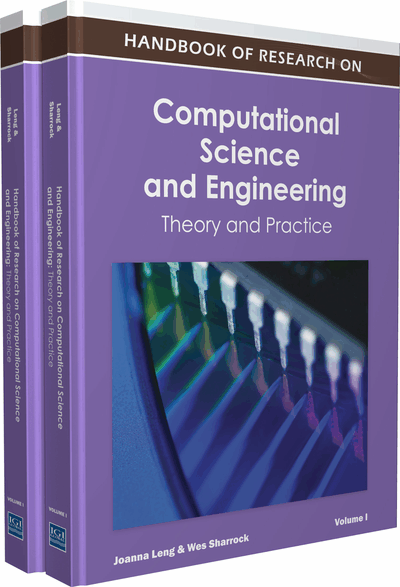# Stochastic Simulations in Systems Biology

Marc Hafner (Ecole Polytechnique Fédérale de Lausanne (EPFL), Switzerland) and Heinz Koeppl (Swiss Federal Institute of Technology Zurich (ETHZ), Switzerland)
DOI: 10.4018/978-1-61350-116-0.ch012
Available
\$37.50
No Current Special Offers

## Abstract

With the advances in measurement technology for molecular biology, predictive mathematical models of cellular processes come in reach. A large fraction of such models addresses the kinetics of interaction between biomolecules such as proteins, transcription factors, genes, and messenger RNA. In contrast to classical chemical kinetics – utilizing the reaction-rate equation – the small volume of cellular compartments requires accounting for the stochasticity of chemical kinetics. In this chapter, we discuss methods to generate sample paths of this underlying stochastic process for situations where the well-stirredness or fast-diffusion assumption holds true. We introduce various approximations to exact simulation algorithms that are more efficient in terms of computational complexity. Moreover, we discuss algorithms that account for the multi-scale nature of cellular reaction events.
Chapter Preview
Top

## Introduction

Molecular biology has undergone significant changes in the last decades. With the enormous amount of data generated by the genomic era, the qualitative reasoning about outcomes of experiments showed inherent limitations. Mathematical models of cellular processes, such as signal transduction (Alon, 2007) are becoming an essential aspect of molecular biology. The dynamic interactions between biomolecules are encapsulated in equations of motion, where multiple biochemical parameters determine rates at which biomolecules are synthesized or degraded, at which they associate, dissociate, or are transformed into other biomolecules. Such equations of motion are often systems of ordinary differential equations (ODE) (Hairer et al., 1991), whose state variables are the concentrations of each type of biomolecules – as it is done in classical chemical kinetics (Laidler, 1987). Yet, some cellular components such as transcription factors or mRNA molecules are present in very few copies and the continuous approach of ODEs falls short under these circumstances. Only a discrete approach capturing the stochastic nature of chemical events can properly describe such dynamics (Wilkinson, 2006; McQuarrie, 1967). In this chapter, the formalism and the main algorithms to perform stochastic simulations of such continuous-time Markov jump processes will be explained. Such simulations are concerned with different time and length scales than classical molecular dynamics simulations. The available methods and implementations of the latter are not applicable to capture the reaction dynamics of large ensembles of biomolecules.

The remaining part of the chapter is organized as follows. The next section introduces the traditional law of mass action and the corresponding rate equations. A discussion on the Markov jump process associated with stochastic chemical kinetics follows. Based on that, a Monte Carlo sampling algorithm developed by Gillespie (Gillespie, 2007) along with its many variants is explained. Subsequently, the first approximate algorithm, the τ-leaping method is derived and some algorithmic aspects of it are highlighted. Further assumptions are made in the following section to obtain the chemical Langevin equation and its simulation is briefly discussed. Finally, we draw conclusions and give some outlook regarding this research area in the last section.

### The Law of Mass Action

Chemical reactions, and as a consequence most events occurring in cellular processes, can be represented as transformations of molecular species Si ∈ {S1, …, Sn}. A reaction ℜj, j∈{1,…,m} with a rate constant kj ∈ ℝ+, is written in a general form as

(1) where Rij ∈ ℕ0 is the stoichiometric coefficient for the species Si as a reactant and Pij ∈ ℕ0 its coefficient as a product (Heinrich and Schuster, 1996).

In the most simplified approach, all reactions of a process follow the law of mass action, originally proposed by Waage and Guldberg (1864): the rate of a reaction is the product of the concentration of the reactants and a constant. Thus the mass action rate functionof reaction ℜj is given by

(2) with k ≡ (k1, …, km)T and where the concentration of each species Si is denoted as zi(t), i∈{1,…,n}. The state of the system is thus the time function.

With the stoichiometric matrix N ∈ ℤn×m, the element of which are Nij = PijRij, that represents the net effect of reaction ℜj on the species Si, the reaction rate equation can be written in vectorial form as

(3)

In case of reactions that do not follow mass action kinetics (i.e. simplifications as Michaelis-Menten (Heinrich and Schuster, 1996)), equation (3) remains valid, but the reaction rate function cannot be expressed as equation (2).

## Key Terms in this Chapter

Michaelis-Menten Rate Equation: Michaelis-Menten kinetics is a model of enzyme kinetics whose rate equation describes the rates of irreversible enzymatic reactions.

Molecular Noise: stochastic fluctuations inherent to biochemical processes due to the discreteness of molecules and their random collision.

SSA: generic term that stands for stochastic simulation algorithms and describes the different implementations of Gillespie’s algorithm (exact methods).

Gaussian (or Normal) Distribution: a continuous probability distribution that describe real-valued random variables that cluster around a mean value. Note that, through the Central theorem, the Poisson distribution with parameter ? is approximately a normal distribution with mean and standard deviation ? for large values of ?.

Hybrid Simulations: simulations where part of the system is evolved using an SSA and the other part is evolved using SDEs or ODEs.

Poisson Distribution: a discrete probability distribution that expresses the probability of a number of events occurring in a fixed period of time if these events independently occur with a known average rate.

## Complete Chapter List

Search this Book:
Reset